# Reflection and refraction

22 de Mar de 2013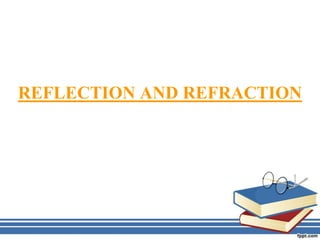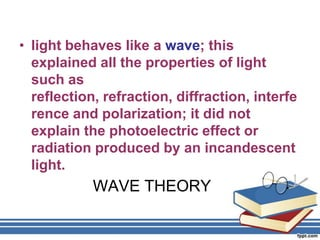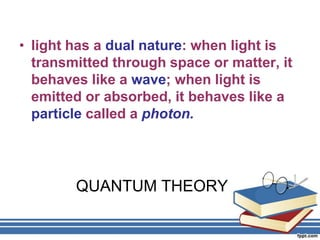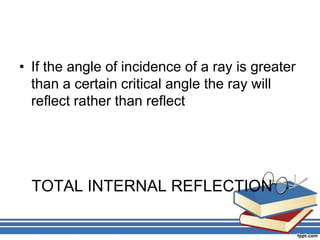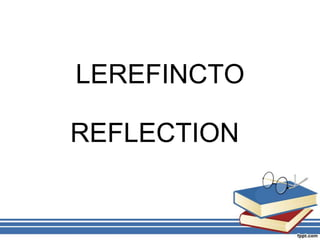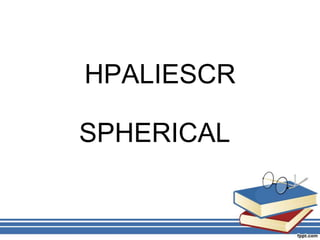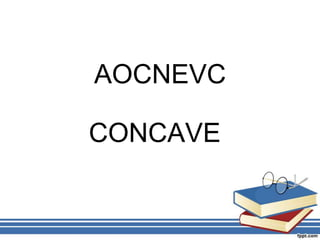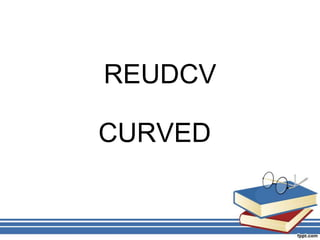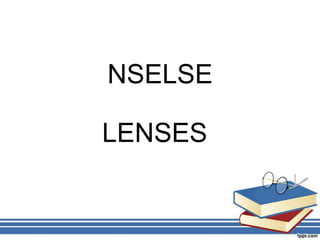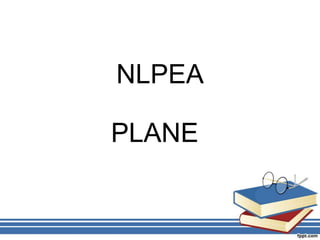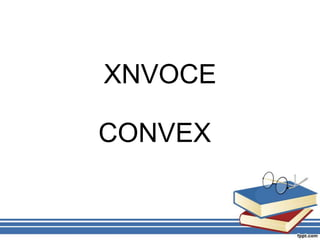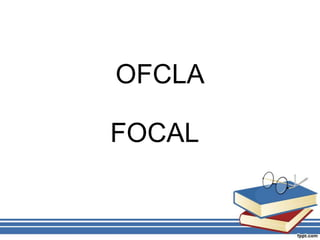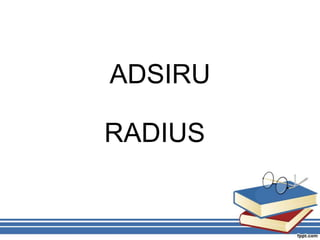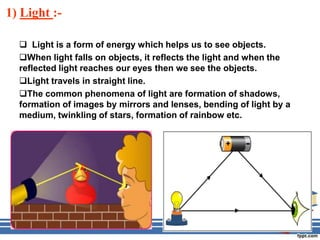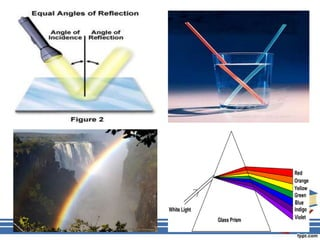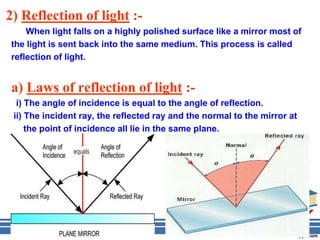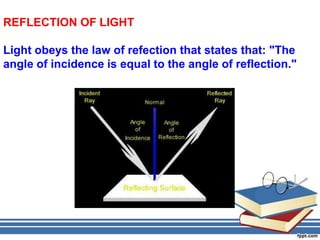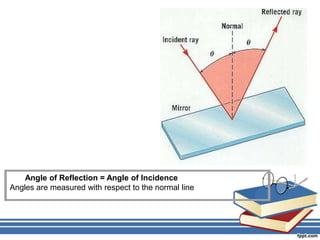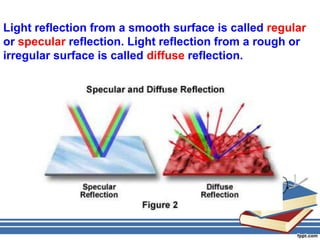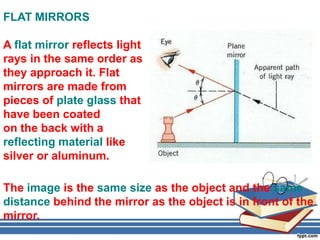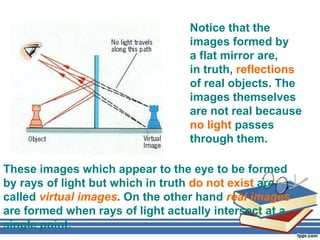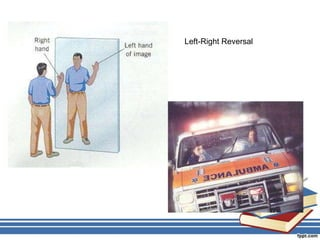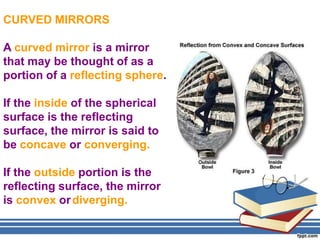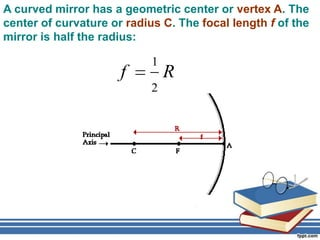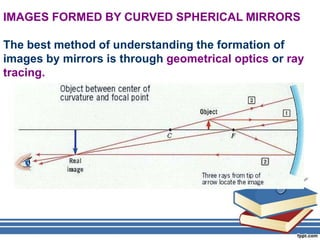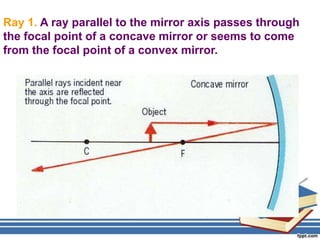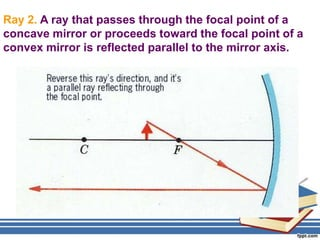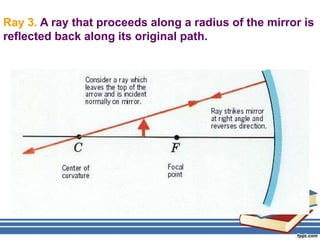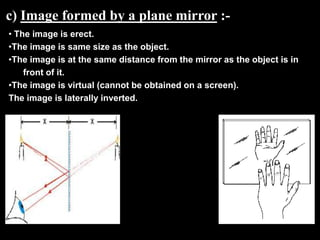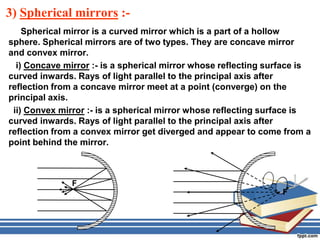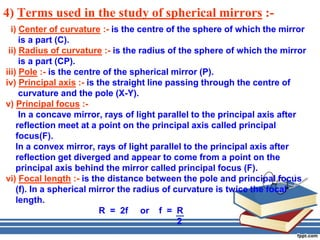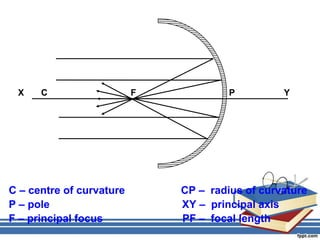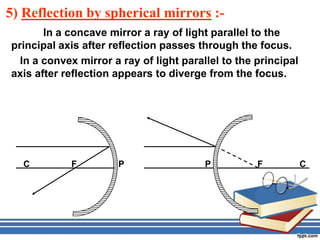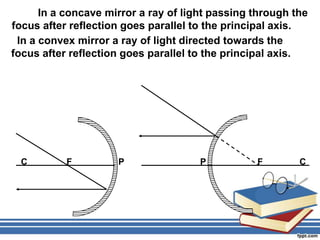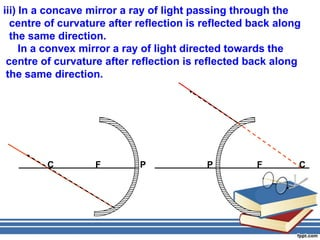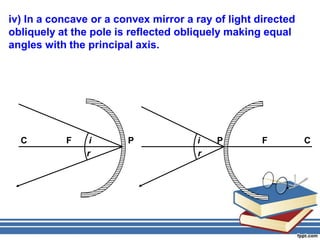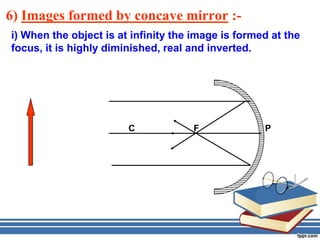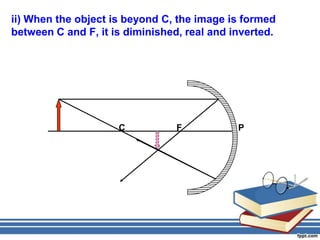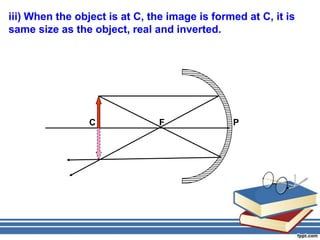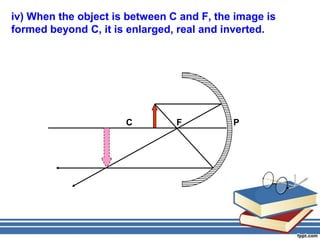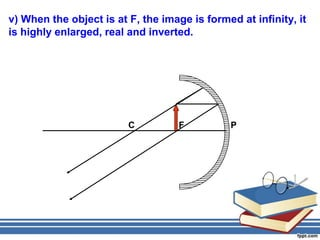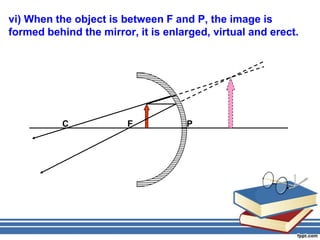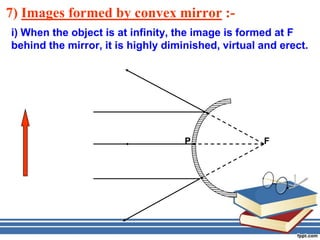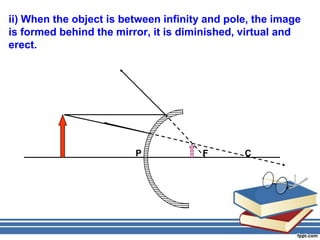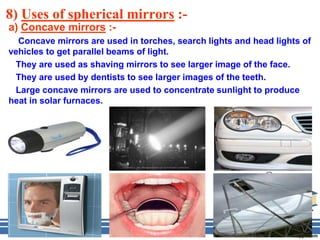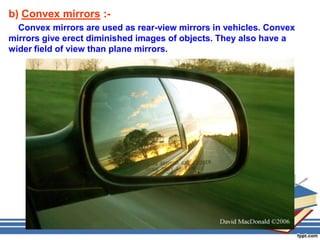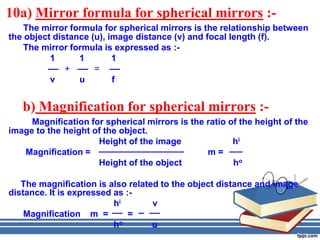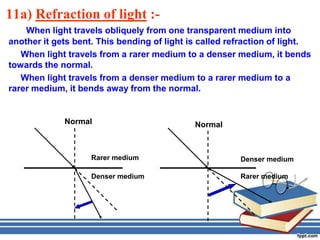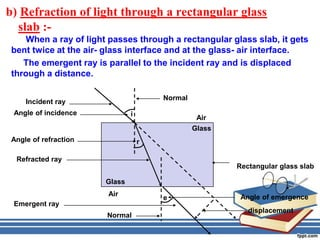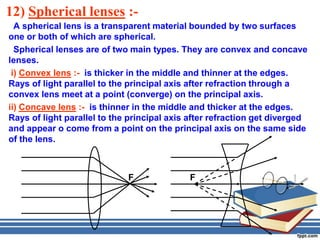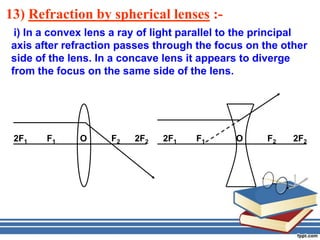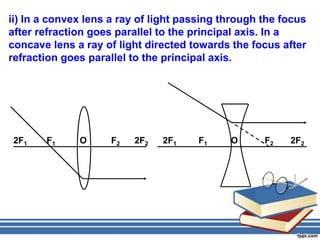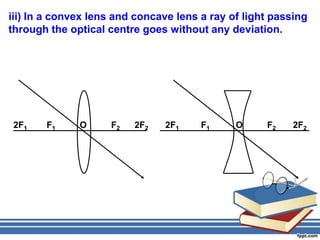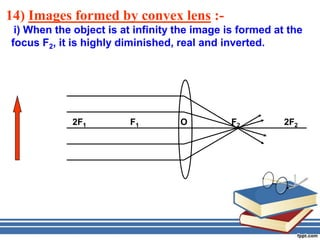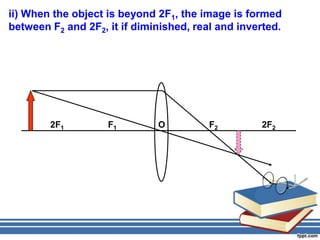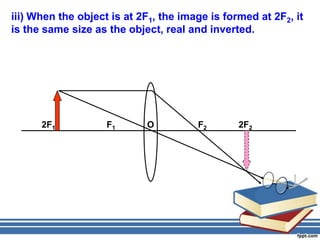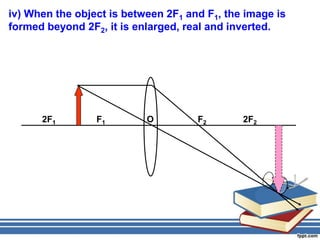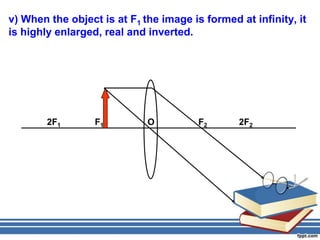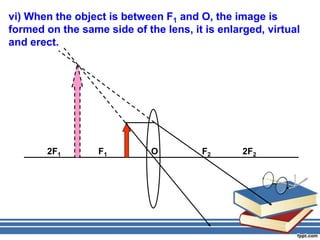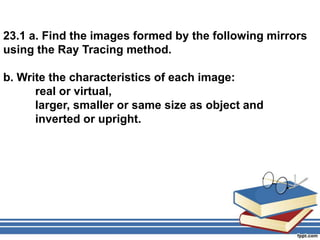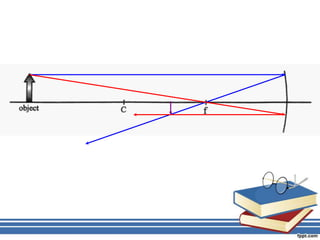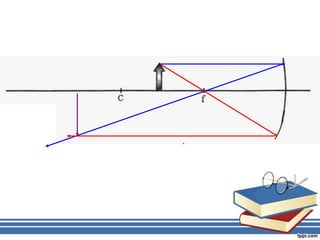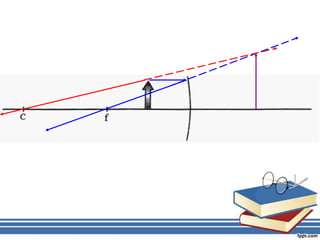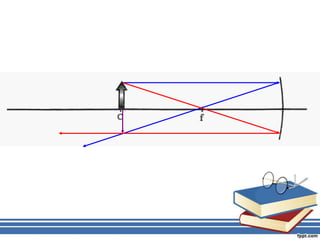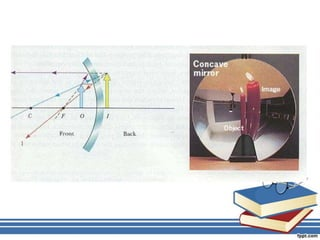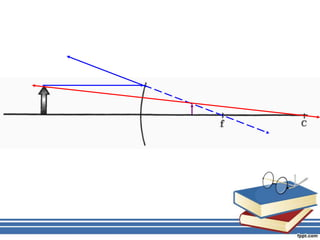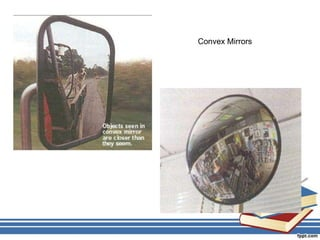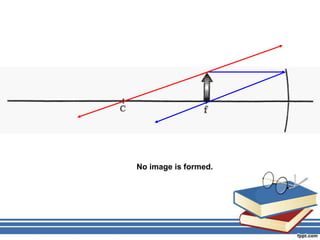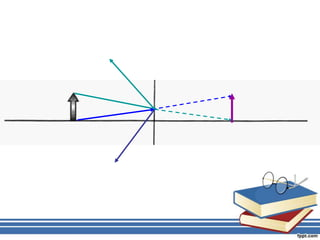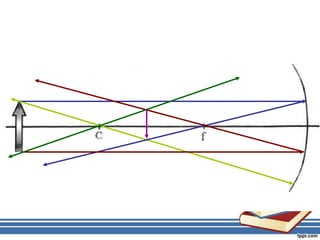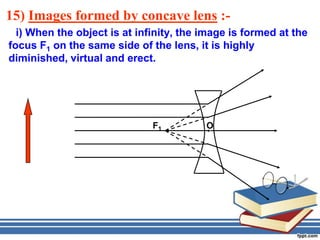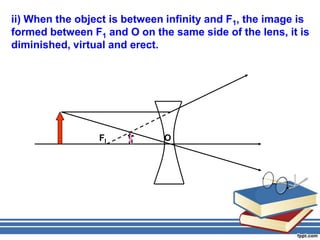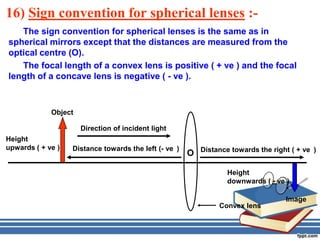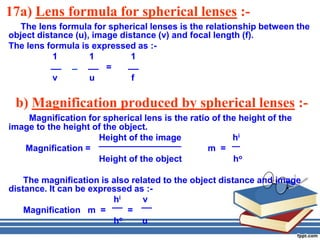1 de 75

### Reflection and refraction

• 2. • light behaves like a wave; this explained all the properties of light such as reflection, refraction, diffraction, interfe rence and polarization; it did not explain the photoelectric effect or radiation produced by an incandescent light. WAVE THEORY
• 3. • light has a dual nature: when light is transmitted through space or matter, it behaves like a wave; when light is emitted or absorbed, it behaves like a particle called a photon. QUANTUM THEORY
• 4. • If the angle of incidence of a ray is greater than a certain critical angle the ray will reflect rather than reflect TOTAL INTERNAL REFLECTION
• 15. 1) Light :-  Light is a form of energy which helps us to see objects. When light falls on objects, it reflects the light and when the reflected light reaches our eyes then we see the objects. Light travels in straight line. The common phenomena of light are formation of shadows, formation of images by mirrors and lenses, bending of light by a medium, twinkling of stars, formation of rainbow etc.
• 17. 2) Reflection of light :- When light falls on a highly polished surface like a mirror most of the light is sent back into the same medium. This process is called reflection of light. a) Laws of reflection of light :- i) The angle of incidence is equal to the angle of reflection. ii) The incident ray, the reflected ray and the normal to the mirror at the point of incidence all lie in the same plane.
• 18. REFLECTION OF LIGHT Light obeys the law of refection that states that: "The angle of incidence is equal to the angle of reflection."
• 19. Angle of Reflection = Angle of Incidence Angles are measured with respect to the normal line
• 20. Light reflection from a smooth surface is called regular or specular reflection. Light reflection from a rough or irregular surface is called diffuse reflection.
• 21. FLAT MIRRORS A flat mirror reflects light rays in the same order as they approach it. Flat mirrors are made from pieces of plate glass that have been coated on the back with a reflecting material like silver or aluminum. The image is the same size as the object and the same distance behind the mirror as the object is in front of the mirror.
• 22. Notice that the images formed by a flat mirror are, in truth, reflections of real objects. The images themselves are not real because no light passes through them. These images which appear to the eye to be formed by rays of light but which in truth do not exist are called virtual images. On the other hand real images are formed when rays of light actually intersect at a single point.
• 24. CURVED MIRRORS A curved mirror is a mirror that may be thought of as a portion of a reflecting sphere. If the inside of the spherical surface is the reflecting surface, the mirror is said to be concave or converging. If the outside portion is the reflecting surface, the mirror is convex or diverging.
• 25. A curved mirror has a geometric center or vertex A. The center of curvature or radius C. The focal length f of the mirror is half the radius: 1 f R 2
• 26. IMAGES FORMED BY CURVED SPHERICAL MIRRORS The best method of understanding the formation of images by mirrors is through geometrical optics or ray tracing.
• 27. Ray 1. A ray parallel to the mirror axis passes through the focal point of a concave mirror or seems to come from the focal point of a convex mirror.
• 28. Ray 2. A ray that passes through the focal point of a concave mirror or proceeds toward the focal point of a convex mirror is reflected parallel to the mirror axis.
• 29. Ray 3. A ray that proceeds along a radius of the mirror is reflected back along its original path.
• 30. c) Image formed by a plane mirror :- • The image is erect. •The image is same size as the object. •The image is at the same distance from the mirror as the object is in front of it. •The image is virtual (cannot be obtained on a screen). The image is laterally inverted.
• 31. 3) Spherical mirrors :- Spherical mirror is a curved mirror which is a part of a hollow sphere. Spherical mirrors are of two types. They are concave mirror and convex mirror. i) Concave mirror :- is a spherical mirror whose reflecting surface is curved inwards. Rays of light parallel to the principal axis after reflection from a concave mirror meet at a point (converge) on the principal axis. ii) Convex mirror :- is a spherical mirror whose reflecting surface is curved inwards. Rays of light parallel to the principal axis after reflection from a convex mirror get diverged and appear to come from a point behind the mirror. F F
• 32. 4) Terms used in the study of spherical mirrors :- i) Center of curvature :- is the centre of the sphere of which the mirror is a part (C). ii) Radius of curvature :- is the radius of the sphere of which the mirror is a part (CP). iii) Pole :- is the centre of the spherical mirror (P). iv) Principal axis :- is the straight line passing through the centre of curvature and the pole (X-Y). v) Principal focus :- In a concave mirror, rays of light parallel to the principal axis after reflection meet at a point on the principal axis called principal focus(F). In a convex mirror, rays of light parallel to the principal axis after reflection get diverged and appear to come from a point on the principal axis behind the mirror called principal focus (F). vi) Focal length :- is the distance between the pole and principal focus (f). In a spherical mirror the radius of curvature is twice the focal length. R = 2f or f = R 2
• 33. X C F P Y C – centre of curvature CP – radius of curvature P – pole XY – principal axis F – principal focus PF – focal length
• 34. 5) Reflection by spherical mirrors :- In a concave mirror a ray of light parallel to the principal axis after reflection passes through the focus. In a convex mirror a ray of light parallel to the principal axis after reflection appears to diverge from the focus. C F P P F C
• 35. In a concave mirror a ray of light passing through the focus after reflection goes parallel to the principal axis. In a convex mirror a ray of light directed towards the focus after reflection goes parallel to the principal axis. C F P P F C
• 36. iii) In a concave mirror a ray of light passing through the centre of curvature after reflection is reflected back along the same direction. In a convex mirror a ray of light directed towards the centre of curvature after reflection is reflected back along the same direction. C F P P F C
• 37. iv) In a concave or a convex mirror a ray of light directed obliquely at the pole is reflected obliquely making equal angles with the principal axis. C F i P i P F C r r
• 38. 6) Images formed by concave mirror :- i) When the object is at infinity the image is formed at the focus, it is highly diminished, real and inverted. C F P
• 39. ii) When the object is beyond C, the image is formed between C and F, it is diminished, real and inverted. C F P
• 40. iii) When the object is at C, the image is formed at C, it is same size as the object, real and inverted. C F P
• 41. iv) When the object is between C and F, the image is formed beyond C, it is enlarged, real and inverted. C F P
• 42. v) When the object is at F, the image is formed at infinity, it is highly enlarged, real and inverted. C F P
• 43. vi) When the object is between F and P, the image is formed behind the mirror, it is enlarged, virtual and erect. C F P
• 44. 7) Images formed by convex mirror :- i) When the object is at infinity, the image is formed at F behind the mirror, it is highly diminished, virtual and erect. P F
• 45. ii) When the object is between infinity and pole, the image is formed behind the mirror, it is diminished, virtual and erect. P F C
• 46. 8) Uses of spherical mirrors :- a) Concave mirrors :- Concave mirrors are used in torches, search lights and head lights of vehicles to get parallel beams of light. They are used as shaving mirrors to see larger image of the face. They are used by dentists to see larger images of the teeth. Large concave mirrors are used to concentrate sunlight to produce heat in solar furnaces.
• 47. b) Convex mirrors :- Convex mirrors are used as rear-view mirrors in vehicles. Convex mirrors give erect diminished images of objects. They also have a wider field of view than plane mirrors.
• 48. 10a) Mirror formula for spherical mirrors :- The mirror formula for spherical mirrors is the relationship between the object distance (u), image distance (v) and focal length (f). The mirror formula is expressed as :- 1 1 1 + = v u f b) Magnification for spherical mirrors :- Magnification for spherical mirrors is the ratio of the height of the image to the height of the object. Height of the image hi Magnification = m= Height of the object ho The magnification is also related to the object distance and image distance. It is expressed as :- hi v Magnification m = = ho u
• 49. 11a) Refraction of light :- When light travels obliquely from one transparent medium into another it gets bent. This bending of light is called refraction of light. When light travels from a rarer medium to a denser medium, it bends towards the normal. When light travels from a denser medium to a rarer medium to a rarer medium, it bends away from the normal. Normal Normal Rarer medium Denser medium Denser medium Rarer medium
• 50. b) Refraction of light through a rectangular glass slab :- When a ray of light passes through a rectangular glass slab, it gets bent twice at the air- glass interface and at the glass- air interface. The emergent ray is parallel to the incident ray and is displaced through a distance. Normal Incident ray Angle of incidence i Air Glass Angle of refraction r Refracted ray Rectangular glass slab Glass Air Angle of emergence e Emergent ray displacement Normal
• 51. 12) Spherical lenses :- A spherical lens is a transparent material bounded by two surfaces one or both of which are spherical. Spherical lenses are of two main types. They are convex and concave lenses. i) Convex lens :- is thicker in the middle and thinner at the edges. Rays of light parallel to the principal axis after refraction through a convex lens meet at a point (converge) on the principal axis. ii) Concave lens :- is thinner in the middle and thicker at the edges. Rays of light parallel to the principal axis after refraction get diverged and appear o come from a point on the principal axis on the same side of the lens. F F
• 52. 13) Refraction by spherical lenses :- i) In a convex lens a ray of light parallel to the principal axis after refraction passes through the focus on the other side of the lens. In a concave lens it appears to diverge from the focus on the same side of the lens. 2F1 F1 O F2 2F2 2F1 F1 O F2 2F2
• 53. ii) In a convex lens a ray of light passing through the focus after refraction goes parallel to the principal axis. In a concave lens a ray of light directed towards the focus after refraction goes parallel to the principal axis. 2F1 F1 O F2 2F2 2F1 F1 O F2 2F2
• 54. iii) In a convex lens and concave lens a ray of light passing through the optical centre goes without any deviation. 2F1 F1 O F2 2F2 2F1 F1 O F2 2F2
• 55. 14) Images formed by convex lens :- i) When the object is at infinity the image is formed at the focus F2, it is highly diminished, real and inverted. 2F1 F1 O F2 2F2
• 56. ii) When the object is beyond 2F1, the image is formed between F2 and 2F2, it if diminished, real and inverted. 2F1 F1 O F2 2F2
• 57. iii) When the object is at 2F1, the image is formed at 2F2, it is the same size as the object, real and inverted. 2F1 F1 O F2 2F2
• 58. iv) When the object is between 2F1 and F1, the image is formed beyond 2F2, it is enlarged, real and inverted. 2F1 F1 O F2 2F2
• 59. v) When the object is at F1 the image is formed at infinity, it is highly enlarged, real and inverted. 2F1 F1 O F2 2F2
• 60. vi) When the object is between F1 and O, the image is formed on the same side of the lens, it is enlarged, virtual and erect. 2F1 F1 O F2 2F2
• 61. 23.1 a. Find the images formed by the following mirrors using the Ray Tracing method. b. Write the characteristics of each image: real or virtual, larger, smaller or same size as object and inverted or upright.
• 69. No image is formed.
• 72. 15) Images formed by concave lens :- i) When the object is at infinity, the image is formed at the focus F1 on the same side of the lens, it is highly diminished, virtual and erect. F1 O
• 73. ii) When the object is between infinity and F1, the image is formed between F1 and O on the same side of the lens, it is diminished, virtual and erect. FI O
• 74. 16) Sign convention for spherical lenses :- The sign convention for spherical lenses is the same as in spherical mirrors except that the distances are measured from the optical centre (O). The focal length of a convex lens is positive ( + ve ) and the focal length of a concave lens is negative ( - ve ). Object Direction of incident light Height upwards ( + ve ) Distance towards the left (- ve ) Distance towards the right ( + ve ) O Height downwards ( - ve ) Image Convex lens
• 75. 17a) Lens formula for spherical lenses :- The lens formula for spherical lenses is the relationship between the object distance (u), image distance (v) and focal length (f). The lens formula is expressed as :- 1 1 1 = v u f b) Magnification produced by spherical lenses :- Magnification for spherical lens is the ratio of the height of the image to the height of the object. Height of the image hi Magnification = m = Height of the object ho The magnification is also related to the object distance and image distance. It can be expressed as :- hi v Magnification m = = ho u